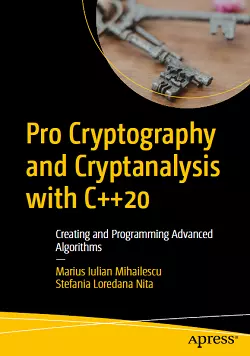# Pro Cryptography and Cryptanalysis with C++20### eBook Details:

• Paperback: 486 pages
• Publisher: WOW! eBook (January 31, 2021)
• Language: English
• ISBN-10: 1484265858
• ISBN-13: 978-1484265857

### eBook Description:

Pro Cryptography and Cryptanalysis with C++20: Creating and Programming Advanced Algorithms: A modern treatment of cryptographic mechanisms using C++20 with practical examples

Develop strong skills and a passion for writing cryptography algorithms and security schemes/modules using C++20 and its new features. You will find the right methods of writing advanced cryptographic algorithms (such as, elliptic curve cryptography algorithms, lattice-based cryptography, searchable encryption, and homomorphic encryption), examine internal cryptographic mechanisms, and discover common ways in which the algorithms could be implemented and used correctly in practice.

What You Will Learn

• Understand where and why cryptography is used and how it gets misused
• Discover what modern cryptography algorithms and methods are used for
• Design and implement advanced cryptographic mechanisms
• See how C++20 and its new features are impacting the future of implementing cryptographic algorithms
• Practice the basics of public key cryptography, including ECDSA signatures and more
• Find out how most of the algorithms can be broken

The authors of this Pro Cryptography and Cryptanalysis with C++20 book avoid the complexities of the mathematical background by explaining its mathematical basis in terms that a programmer can easily understand. They show how “bad” cryptography creeps in during implementation and what “good” cryptography should look like. They do so by showing and comparing the advantages and disadvantages based on processing time, execution time, and reliability.